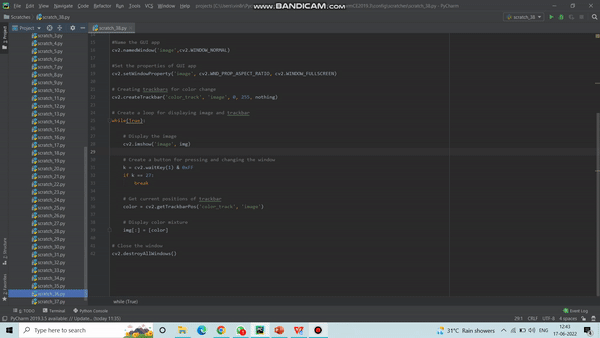# Python OpenCV – setWindowProperty() Function

• Last Updated : 27 Jun, 2022

In this, we are briefly covering the setWindowProperty() function with its working example from the OpenCV package in the python programming language.

Do you want to change the parameters of a window dynamically? Then, you must check out the function setWindowProperty() which enables changing the properties of a window. In this article, we will discuss how to use setWindowProperty() in OpenCV.

Syntax:

cv2.namedWindow(windowsName, prop_value)

cv2.setWindowProperty(windowsName, prop_id, prop_value)

Parameters:

• windowsName: Name of the window
• prop_id: Window property to edit such as cv2.WINDOW_NORMAL, cv2.WINDOW_KEEPRATIO, cv2.WINDOW_FULLSCREEN, etc.
• prop_value: New value of the window property such as cv2.WND_PROP_FULLSCREEN, cv2.WND_PROP_AUTOSIZE, cv2.WND_PROP_ASPECT_RATIO, etc.

## Stepwise Implementation:

### Step 1: First of all, import the libraries OpenCV and NumPy:

In this step, we are simply importing the required libraries to the working python environment to use its functionalities further as per needed.

Here, we are importing the cv2 and the Numpy library, the cv2 is the OpenCV package which helps us to call the setWindowPropoerty() function within the environment, and further, the Numpy package is responsible for the random mathematical operations.

Syntax:

```import cv2
import numpy```

### Step 2: Now, create a function nothing for creating the trackbar:

In this step, we will create a function titled nothing which can be used as a callback function for the trackbar.

Below, we have created a trackbar using createTrackbar() function for which we need to pass some user-defined function according to which createTrackbar() function will perform, thus we have created a nothing function.

```def nothing(x):
pass```

### Step 3: Then, create a window with a black image.

In this step, we return a new array black in color of specified size. Here, the function numpy.zeros() is used which returns a new array of given shape and type.

• shape : integer or sequence of integers
• dtype : Data type of returned array
`img = numpy.zeros((300, 512, 3), numpy.uint8)`

### Step 4: Next, assign the name to your GUI app.

In this step, we have used a namedWindow() function which is used to create a window with a suitable name and size to display images and videos on the screen.

• window_name: Name of the window.
• prop_value: New value of the window property such as cv2.WND_PROP_FULLSCREEN, cv2.WND_PROP_AUTOSIZE, cv2.WND_PROP_ASPECT_RATIO, etc.
`cv2.namedWindow(windowsName, prop_value)`

### Step 5: Set the properties of the GUI app.

In this step, we will use the setWindowProperty() function which changes the parameters of the window dynamically.

• windowsName: Name of the window.
• prop_id: Window property to edit such as cv2.WINDOW_NORMAL, cv2.WINDOW_KEEPRATIO, cv2.WINDOW_FULLSCREEN, etc.
• prop_value: New value of the window property such as cv2.WND_PROP_FULLSCREEN, cv2.WND_PROP_AUTOSIZE, cv2.WND_PROP_ASPECT_RATIO, etc.

cv2.setWindowProperty(windowsName, prop_id, prop_value)

### Step 6: Moreover, create a trackbar for changing the color.

In this step, we have used a createTrackbar() function which is used to create the trackbar in OpenCV.

cv2.createTrackbar(‘color_track’, ‘image’, 0, 255, nothing)

### Step 7: Further, set the maximum value of the trackbar.

In this step, we have used the setTrackbarPos() function that sets the position of the created trackbar in the specified window. This function doesn’t return anything.

cv2.setTrackbarMax(‘color_track’, ‘image’, #Maximum-Value)

### Step 8: Later on, create a loop for displaying the image and trackbar.

In this step, we create a while loop which runs continuously until the user presses the Escafe button.

`while(True):`

Step 8.1: Next, display the image.

In this step, we will show the black image which you created earlier in step-3.

`  cv2.imshow('image', img)`

Step 8.2: Then, create a button for pressing and changing the window.

In this step, we use waitkey to add delay and stop the function when the user presses ‘Escafe’ key.

```  k = cv2.waitKey(1) & 0xFF
if k == 27:
break```

Step 8.3: Furthermore, get the current positions of the trackbar.

In this step, we have used a getTrackbarPos() function that returns the current position of the specified trackbar. This function takes two arguments for which the first is the trackbar name and the second one is the window name which is the parent of the trackbar.

`  color = cv2.getTrackbarPos('color_track', 'image')`

Step 8.4: Now, display the color mixture.

In this step, we will change the color of black image according to the trackbar. The color of image changes from black to white.

`  img[:] = [color]`

### Step 9: Finally, close and destroy all the windows.

In this step, we will finally close all the windows we have opened till now. It does not take any parameters and doesn’t return anything.

`cv2.destroyAllWindows()`

## Python3

 `# Python program for setWindowProperty() ` `#Python OpenCV ` ` `  `# Importing the libraries OpenCV and numpy ` `import` `cv2 ` `import` `numpy ` ` `  `# Create a function 'nothing' for creating trackbar ` `def` `nothing(x): ` `    ``pass` ` `  `# Creating a window with black image ` `img ``=` `numpy.zeros((``300``, ``512``, ``3``), numpy.uint8) ` ` `  `#Name the GUI app ` `cv2.namedWindow(``'image'``,cv2.WINDOW_NORMAL) ` ` `  `#Set the properties of GUI app ` `cv2.setWindowProperty(``'image'``, cv2.WND_PROP_ASPECT_RATIO, ` `                      ``cv2.WINDOW_FULLSCREEN) ` ` `  `# Creating trackbars for color change ` `cv2.createTrackbar(``'color_track'``, ``'image'``, ``0``, ``255``, nothing) ` ` `  `# Create a loop for displaying image and trackbar ` `while``(``True``): ` ` `  `    ``# Display the image ` `    ``cv2.imshow(``'image'``, img) ` ` `  `    ``# Create a button for pressing and changing ` `    ``# the window ` `    ``k ``=` `cv2.waitKey(``1``) & ``0xFF` `    ``if` `k ``=``=` `27``: ` `        ``break` ` `  `    ``# Get current positions of trackbar ` `    ``color ``=` `cv2.getTrackbarPos(``'color_track'``, ``'image'``) ` ` `  `    ``# Display color mixture ` `    ``img[:] ``=` `[color] ` ` `  `# Close the window ` `cv2.destroyAllWindows()`

Output:My Personal Notes arrow_drop_up
Recommended Articles
Page :